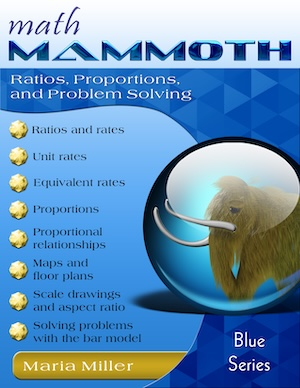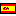GIVEAWAY! Win a Math Mammoth bundle or a grade level of your choice (13 winners!). Take part here. The giveaway runs through Sep 30, 2023.
^
You are here: HomeBlue Series → Ratios, Proportions & Problem Solving

# Math Mammoth Ratios, Proportions & Problem Solving134 pages

## Prices & ordering

Printed copy \$11.90

(credit cards, Google Pay, Paypal, PO)Este libro en español

Math Mammoth Ratios, Proportions & Problem Solving is a worktext that concentrates, first of all, on two important concepts: ratios and proportions, and then on problem solving. The book is best suited for grade levels 6 and 7.

The book starts out with the basic concepts of ratio, rate, and unit rate. The lessons on unit rates not only show how to calculate them and gives practice at doing so, but also include practice with unit rates that involve complex fractions.

The two lessons on equivalent rates allow students to solve a variety of word problems involving ratios and rates. We also connect the concept of rates (specifically, tables of equivalent rates) with ordered pairs, use equations (such as y = 3x) to describe these tables, and plot the ordered pairs in the coordinate plane. Then, students encounter the concept of aspect ratio, which is simply the ratio of a rectangle's width to its height, and solve a variety of problems involving aspect ratio.

Next, students learn how rates can be used to convert measurement units. This method is in addition to the methods for converting measurement units that are explained in Math Mammoth books on decimals. It does not mean that students should "change over" and forget what they have learned earlier — it is simply a different method for doing the conversions. Some students may choose one method over another; some may be able to master all of the methods. Most will probably choose one method they prefer for doing these conversions.

After this, we turn our attention to proportions (which is an equation of two ratios). The lessons show how to solve proportions using cross-multiplying and how to set up proportions the correct way. We also study briefly what cross-multiplying is based on (it is not a magic trick!).

The concept of direct variation is introduced in the lesson Proportional Relationships. Writing and graphing equations gives a visual understanding of proportionality. In two following lessons on proportions, students also practice solving rate problems in different ways, using the various methods that they have learned throughout the book.

The lessons Scaling Figures, Floor Plans, and Maps give useful applications and more practice to master the concepts of proportions. There is also an optional lesson, Significant Digits, that deals with the concept of the accuracy of a measurement and how it limits the accuracy of the solution.

Lastly, we study various kinds of word problems involving ratios and use a bar model to solve these problems. These lessons tie ratios in with the student's previous knowledge of bar models as a tool for problem solving.

The PDF version of this book can be filled in on a computer, phone, or tablet, using the annotation tools found in many PDF apps. See more.

## Better Yet - Bundle Deals!

*BONUS*: Buy any bundle listed above, and get the fully upgraded Soft-Pak software for FREE (includes 4 math and 2 language arts programs, complete with on screen and printable options).

## How and where to order

You can buy Math Mammoth books at:

• Here at MathMammoth.com website — simply use the "Add to cart" buttons you see on the product pages.
• Rainbow Resource carries printed copies for the Light Blue series books, plus several CDs (Light Blue and Blue series).
• Homeschool Buyers Club offers download versions of the Light Blue series, plus the Blue series bundle.
• Homeschool Planet sells the digital versions of the main curriculum and lesson plans to go with them.
• Lulu sells printed copies for most of the Math Mammoth materials (various series).

By purchasing any of the books, permission IS granted for the teacher (or parent) to reproduce this material to be used with his/her students in a teaching situation; not for commercial resale. However, you are not permitted to share the material with another teacher.

In other words, you are permitted to make copies for the students/children you are teaching, but not for other teachers' usage.

Math Mammoth books are PDF files. I recommend you use Adobe Reader to view them, including if you use a Mac. You can try other PDF viewers, but they may at times either omit or mess up some of the images.
WAIT!

Receive my monthly collection of math tips & resources directly in your inbox — and get a FREE Math Mammoth book!You can unsubscribe at any time.

### Math Mammoth Tour

Confused about the different options? Take a virtual email tour around Math Mammoth! You'll receive:

An initial email to download your GIFT of over 400 free worksheets and sample pages from my books. Six other "TOURSTOP" emails that explain the important things and commonly asked questions concerning Math Mammoth curriculum. (Find out the differences between all these different-colored series!)

This way, you'll have time to digest the information over one or two weeks, plus an opportunity to ask me personally about the curriculum.
A monthly collection of math teaching tips & Math Mammoth updates (unsubscribe any time)### "Mini" Math Teaching Course

This is a little "virtual" 2-week course, where you will receive emails on important topics on teaching math, including:

- How to help a student who is behind
- Troubles with word problems
- Teaching multiplication tables
- Why fractions are so difficult
- The value of mistakes
- Should you use timed tests
- And more!

A GIFT of over 400 free worksheets and sample pages from my books right in the very beginning.A monthly collection of math teaching tips & Math Mammoth updates (unsubscribe any time)
Enter your email to receive math teaching tips, resources, Math Mammoth news & sales, humor, and more! I tend to send out these tips about once monthly, near the beginning of the month, but occasionally you may hear from me twice per month (and sometimes less often).• A GIFT of over 400 free worksheets and sample pages from my books.